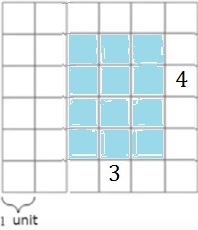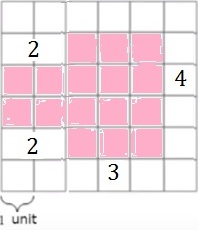# Perimeter and Area on a Grid

A grid is made of a number of unit squares. Figures are drawn on a grid.
The perimeter of a figure is the total length of its outline or boundary and this is found by counting the number of unit lengths along the boundary of the figure. The area of a figure on grid is found by counting the total number of unit squares it occupies.

Find the perimeter and the area of the shaded shape on the grid.### Solution

Step 1:

The shaded shape on the grid is a rectangle. Its length is 4 units and width is 3 units.

Step 2:

Perimeter of the shaded shape = 3 + 4 + 3 + 4 = 14 units

Step 3:

Area of the shaded shape = length × width

= 4 × 3 = 12 square units

Find the perimeter and area of the shaded shape on the grid.### Solution

Step 1:

The shaded shape on the grid is a combination of shapes. Its side lengths are 2, 2, 2, 1, 3, 4, 3, and 1 units

Step 2:

Perimeter of the shaded shape = 2 + 2 + 2 + 1 + 3 + 4 + 3 + 1 = 18 units

Step 3:

Area of the shaded shape = sum of shaded squares × 1 square unit

= 16 × 1 = 16 square units